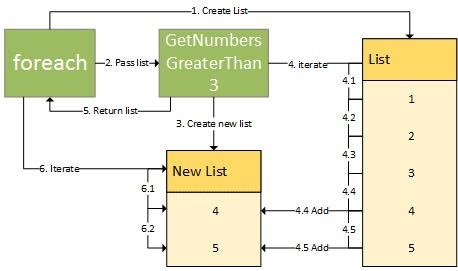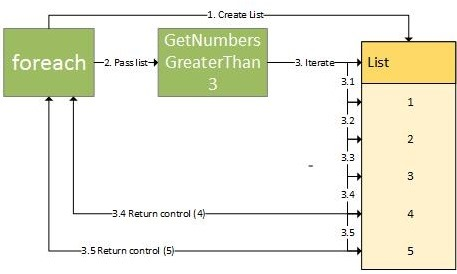# 迭代器示例

IEnumerable<int> GetNumbers()
{
yield return 1;
yield return 2;
yield return 3;
}

foreach (var item in GetNumbers())
{
Console.WriteLine(item);
}


# 传统循环 vs 迭代器

IEnumerable<int> GenerateWithoutYield()
{
var i = 0;
var list = new List<int>();
while (i<5)
return list;
}
foreach(var number in GenerateWithoutYield())
Console.WriteLine(number);


1. GenerateWithoutYield 被调用
2. 整个方法执行完毕并且返回构建好的数字列表
3. foreach 遍历列表中的所有值然后输出

IEnumerable<int> GenerateWithYield()
{
var i = 0;
while (i<5)
yield return ++i;
}
foreach(var number in GenerateWithYield())
Console.WriteLine(number);


1. GenerateWithYield() 被调用
2. 方法返回 IEnumerable<int> ，这里返回的并不是一个list，而是一个类似状态机运行机制的迭代器。以上代码中的 GenerateWithYield() 会返回5个数字，但并不是一次执行完毕。而是每次请求的时候逐一返回。
3. foreach 循环调用 GenerateWithYield() 知道所有数字输出完毕

# 无限循环

// 迭代器方法
IEnumerable<int> GenerateWithYield()
{
var i = 0;
while (true)
yield return ++i;
}

// 传统方法
IEnumerable<int> GenerateWithoutYield()
{
var i = 0;
var list = new List<int>();
while (true)
return list;
}

// 打印数字
foreach(var number in GenerateWithoutYield()))
Console.WriteLine(number);

foreach(var number in GenerateWithYield())
Console.WriteLine(number);


# 实际用途

## 自定义迭代

IEnumerable<int> GetNumbersGreaterThan3(List<int> numbers)
{
var theNumbers = new List<int>();
foreach(var nr in numbers)
{
if(nr > 3)
}
return theNumbers;
}
foreach(var nr in GetNumbersGreaterThan3(new List<int> {1,2,3,4,5})
Console.WriteLine(nr);IEnumerable<int> GetNumbersGreaterThan3(List<int> numbers)
{
foreach(var nr in numbers)
{
if(nr > 3)
yield return nr;
}
}
foreach(var nr in GetNumbersGreaterThan3(new List<int> {1,2,3,4,5})
Console.WriteLine(nr);## 状态控制

IEnumerable<int> Totals(List<int> numbers)
{
var total = 0;
foreach(var number in numbers)
{
total += number;
}
}
foreach(var total in Totals(new List<int> {1,2,3,4,5})
Console.WriteLine(total);

// Output： 1    3    6    10    15


## 延迟执行

C# 的LINQ部分就用到了这一特性。示例：

var dollarPrices = FetchProducts().Take(10)
.Select(p => p.CalculatePrice())
.OrderBy(price => price)
.Take(5)
.Select(price => ConvertTo\$(price));


• 获取所有1000个产品
• 计算所有产品的价格
• 对价格进行排序
• 将价格兑换为美元
• 取出价格前5的产品

• 取出10个产品
• 计算取出产品的价格
• 对价格进行排序
• 将5个产品的价格兑换为美元